# Using Neural Network to Classify Clothes and Shoes

Using a Neural Network to Classify Images to classify Images on the Fashion MNIST dataset.

If you are unfamiliar with neural networks, check out this article. It covers the different aspects to conceptually understand how neural networks. Here we are going to be applying this knowledge by actually building a neural network.

Below is a visual of a neural network. The basic structure goes as follows. In neural networks, we have input values (x₁ and x₂). We multiply them by their weights w₁ and w₂. Then we add a bias value to determine the activation value that we want our neuron to have.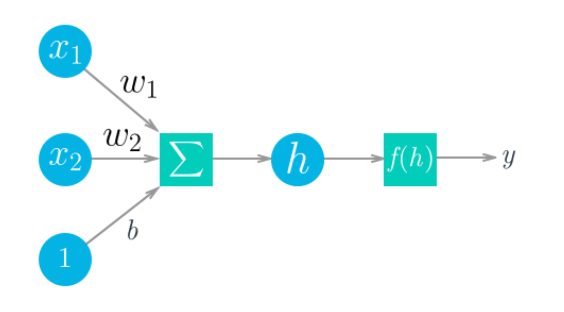Visual process of a neural network

After calculating our weighted sum (w₁ * x₁ + w * x + b) we get h. H is a neuron in the hidden layer of our network. This h value later becomes part of the input to create a value y (our output). To get the value y we need to multiply our weight sum (h) by a function. This function turns our weight sum into a value between 0 and 1, if not our neurons would not be able to function. Below you can what it looks like in the equation form.

The one thing that is important to note in the equation above is Σ called a summation or a sigma. This symbol communicates that we are taking the vector of wᵢ and x to multiply them by each other. The letter i represents how many of these vectors there are. If i =3 then we have (w₁ * x₁) + (w * x) + (w₃ * x₃). This symbol provides a convenient way to write out our vector multiplication. Imagine if i = 1000. Writing all of those vectors out all of those vectors is extremely time-consuming so sigma is used as the general equation.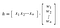Note: this vector multiplication has bias of 0 (don’t forget about the bias.)

The image above is a vector representation of w and x. We would multiply the values by their corresponding number. For example (x1 * w1), (x2 * w2) and (xₙ * wₙ) with n serving the same purpose as i from the previous image.

These vectors can be used to represent a tensor, which is a generalization of matrices. A vector is a 1-dimensional tensor as it only has one path it can operate. A matrix that is a 2-dimensional tensor can operation across the tensor in two ways. From left to right and top to bottom. An array with three indices is a 3-dimensional tensor, you could think of this as an RGB color image for every pixel there is a value for the red, the green, and the blue channels.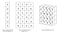1, 2, and 3 dimensional tensors

## Building the Model

In this neural network, I used a framework called PyTorch. PyTorch is an open-source machine learning library used for computer vision and natural language processing. Pytorch, like any other machine learning framework, uses tensors to build its model.

The first thing we are going to do is import torch which is Pytorch. Torchvision allows us to import the Fashion MNIST dataset that we are using. We also need to import helper which is a custom script that you need to download to use. It allows us to see our model’s prediction (probability distribution) of what item it was given.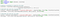Importanting important libraries and the Fashion MNIST dataset

Now import the nn module which is said to provide a more convenient and powerful method for defining network architectures. We also import optim as in optimizers are algorithms used to change the attributes of the neural network such as weights and learning rate to reduce the losses (which is explained later throughout this article.Importing module to build a clean network architecture, optimizer to help in reducing loses when training the model, and functions to be able to have working neurons.

nn.functional is what we use to able to be able to implement a function to turn our weighted sum into a value between 0 and 1 (sigmoid, ReLu) or functions like softmax that helps us create a probability distribution from our model.

In our next step, we need to name our class which can be called anything. For this example, I chose to use “Classifier”. The important idea is we use the subclass nn. module so we can use the __init__ method. This makes Pytorch register all the different layers and operations that we are putting into this network.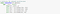Fully connected networks from layer 1 to 4.

The fc1, fc2, fc3…fcₙ is an abbreviated way to say fully connected network. This communicates to the reader that each line moves from one layer to another in the neural network.

nn.Linear takes our input value (ex. fc1 input = 784 neurons) and turns it into the outvalue that we specify (ex. fc1 output = 256 neurons). It does this using a linear transformation (hence nn.Linear) which is our weighted sum

nn.Linear creates an object that creates the parameters for the weights and the biases. When we pass the vector(wᵢ) through the hidden layers it is going to automatically calculate the linear transformation for us. In other words, it takes from 784 neurons (input in fc1) to 265 neurons (output in fc1).

The forward function communicates that this is the feedforward section of our neural network. The x.view(x.shape , -1) makes sure that the input tensor is flattened which means reshapes the input tensor to have a shape that is equal to the number of elements contained in the tensor that is used in the calculations.

Under the forward function, we are taking the different values from the outputs of each layer and putting them into a ReLu (rectified linear unit) function, which is a nonlinear function does the same action as sigmoid of taking the weighted sum and turning it into a number between 0 and 1, but it turns out the ReLu has an easier and faster training process than sigmoid so we used ReLu instead.

We want the output of our network to be a probability distribution that gives us the probability that the image belongs to one of our classes. To create this probability distribution we use the softmax function. Similar to the sigmoid function it squishes each of the input values x between 0 and 1, but the big upside is that it normalizes all the values of the sum of each of the probabilities is 1. This makes it so we can see our accuracy out of 100%, giving us the ability to have a probability distribution.

The Dimension (dim=1)makes it so that it calculates the function across the columns and instead of the rows. The reason we want this to happen is that we have a batch of examples (images from our dataset) that we are passing through our network and each row is one of those examples so, we want to make sure that we’re calculating the softmax function across each of our examples and not across each feature in our batches.

At the start, before we train our network our probability looks like this. Without the training, our network is randomly assigning equal probability to all of our classes because it has not yet seen the data for it to be able to recognize the number.

## How Our Model Starts to Learn.

The machine does this through something called a loss function sometimes also called the cost. It is simply a measure of the prediction error. If we have an image of a skirt and our model predicts it incorrectly, we want to measure how far away our network prediction is from the correct label. This can be done using the loss function.

The loss value depends on the output of our network, which is based on weight (like network parameters). So we can adjust our weights so that our loss is minimized. When we have a low loss value then we know that our network is making as good predictions as it can.

This happens through a process called gradient descent. The gradient is the slope of the loss function. Our gradient always points in the direction of the fastest change.

So imagine you are on the mountain you made it to the top and it is not time to come back down. Your goal is to get off of the mountain as fast as you can. Below are the possible directions you could go.

With the goal of going down as fast as possible you need you will go directly down. It is the same thing with our gradient descent, but for the gradient which points upwards we take the negative of it take us downwards. The further down we go the lower our loss function gets making it our model have a better ability to predict the images that we provide it.

One issue that you can come across is your gradient can be taking steps so large it ends up bouncing around the trough and never reaching the local minimum within the graph (picture on the right side). This is have something called a learning rate. Which is our restriction on how fast the negative gradient can be to ensure it is not bouncing around and hits the local minimum without much difficulty.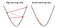Results of different learning rates with gradient descent

Below we see the model, which is just the name of class. NN.NLLLoss gives us our cost and then we use optimizers which are algorithms or methods used to change the attributes of the neural network (weights and learning rate) to reduce the cost in our model.

When we train our network we have epochs or the number of cycles that a neural network has went through training data. The images are being used as the input values. The criterion is calculating our loss.

Our optimizer says zero_grad() because PyTorch by default accumulates gradients that means if you do multiple forward passes (process of going from input to output in a neural network) and multiple backward passes (by using gradient descent to calculate our cost, then using optimizers to reduce our cost), when calculating you are gradients it’s is going to keep summing up those gradients.

If you don’t clear your gradients then you are going to be getting gradients from the previous training step along with the one in your current training step. This ends with your network not training properly, so make sure to you zero_grad().

Then we go through the loss.backward to calculate our gradient and we are taking an optimizer step allowing us to train our model. After only 5 cycles of training, our model was able to confidently predict what they image was.

16 yo TKS innovate wanting to give value to other people

## More from Ahmed Hassan

16 yo TKS innovate wanting to give value to other people